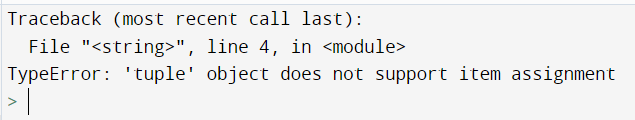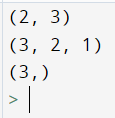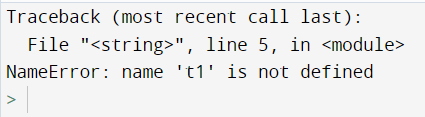# Python Tuples

Tuples in python is an in-built data structure that is efficient we don’t want to modify the contents of a data structure accidentally or intentionally.

A tuple is basically a read-only list i.e. they are immutable. Tuples are represented by parenthesis and items are separated by commas.

Tuples are quite similar to lists in indexing, accessing, etc basic operations. We can’t add a new object or delete an existing object.

Declaration

We just simply create a tuple using parenthesis or directly, python will store the elements as a tuple only.

Code:

## Python Code

``````t1 = (1,2)
t2 = 1,2
print(t1)
# use type() function to get the type of passed arguement
print(type(t2))
``````

Output:

(1, 2)
<class ‘tuple’>

### Concatenation of tuples

Just like the simple concatenation of 2 lists, in python, we can perform the same for the tuples.

Code:

## Python Code

``````letters = ( "a", "b", "c" ,"d" ,"e" )
numbers = (1,2,3,4,5)
combined = letters + numbers
print(combined)
``````

Output: (‘a’, ‘b’, ‘c’, ‘d’, ‘e’, 1, 2, 3, 4, 5)

We can use * operator to repeat tuples.

Code:

## Python Code

``````numbers = (1,2,3,4,5)
num2=numbers * 2
print(num2)
``````

output: (1, 2, 3, 4, 5, 1, 2, 3, 4, 5)

Also, we can convert a list into a tuple using the tuple( iterable ) function which takes an iterable as an argument and we know that lists are iterable in python.

Code:

## Python Code

``````list1=[1,2,3]
t1=tuple(list1)
print(t1)
``````

Output: (1, 2, 3)

Immutable Tuples

To show whether tuples are mutable or not, let us execute the following code:

Code:

## Python Code

``````t1 = (1, 2, 3)
t1 = 8
print(t1)
``````

Output:Slicing Tuples

The tuples are also available for slicing and can be done in similar ways to what we can do in lists. Let us see some examples:

Code:

## Python Code

``````t1 = (1, 2, 3)
print(t1[1:])
print(t1[::-1])
print(t1[2:3])
``````

Output:Deleting a tuple

Unlike lists, a fraction of the tuple can’t be deleted. We have to completely delete the tuple using the del available in python.

Code:

## Python Code

``````t1=(1,2,3,5)
del t1
print(t1)
``````

Output: